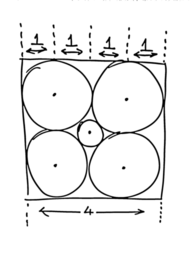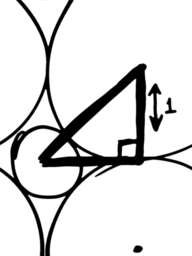# Spikey SpheresSubscribe!My latest posts can be found here:
Previous blog posts:
I've recently been working on an optimisation problem, and I've come to realise that I can consider it as wandering around on a smooth landscape in 1800 dimensions. Strange number, I know, but that's the way it's worked out.

 A word of warning ... I'm presenting here something called an analogy. It's not perfect, it has problems, it's not exact, but there are ways in which it can aid your thinking. I'm giving a way of visualising high-dimensional spheres, a way that has its limitations, but which can help avoid making mistakes based on your direct, 3D experience. It's an aid, nothing more.
The problem is that the usual visualisation of hill-climbing, or hill-descending, is that you're on a gently undulating vista, and that some directions are up, some are down, and it's easy to decide which is which. You write the code, set off, and somehow the system never finds a good solution.

Part of the problem is that there is simply a lot of space to explore. If you discretise space and have 1000 places to be in each dimension, 2 dimensions gives you a million places to be. That's not so bad. 1800 dimensions gives you ${10}^{5400}$ places to be. That's not good. You definitely need to move in moderately large strides, and then hone your solution by using binary chop or similar techniques.

But it's worse than that. The problem is that the error function may be "smooth," but your intuition of what this means is wrong.

Let's give you a different intuition. This isn't complete, and it certainly isn't perfect, but it can help to understand the problem.Figure 1Figure 2
Take four unit circles and arrange them in a square. (Figure 1 at right) Enclose them in a 4x4 box, so that each circle touches both two other circles, and two sides of the bounding square. (I know the diagram is rubbish - I'm not Giotto, and I'm writing this on the move.)

There's a small gap in the middle, and in it we can put a circle touching all four of the surrounding circles.

How big is it?

By Pythagoras, the distance from the centre of the bounding square (and hence of the central circle) to the centre of one of the other circles is $\sqrt{2}$ (Figure 2 at right)

The big circles have radius 1, so the smaller circle has radius $\sqrt{2}-1$ which is about 0.4142, a bit more than 0.4.

If we go to three dimensions we get a 4x4x4 cube with 8 spheres in it. A sphere of size 0.4 fits between the spheres on a face, and pushing it into the centre means it can rattle around. Now we can grow it until it touches all eight spheres..

How big?

The distance from the centre of the cube to the centre of a sphere is $\sqrt{3}.$ Subtracting off the radius of one of the corner spheres we get a radius for the internal sphere of $\sqrt{3}-1,$ which is about 0.732.

More generally, in "n" dimensions the distance from the centre of the hyper-cube to the centre of a hyper-sphere is $\sqrt{n},$ and so the radius of the central hyper-sphere is $\sqrt{n}-1.$

(I'm already tired of writing "hyper" - I'll leave it out from now on)

 It's always worth checking an extreme case. When $n$ is 1, the formula predicts a central "sphere" of size 0, and that's right. In the one-dimensional case we have a line of length 4, and the "spheres" at each "corner" - remember they are radius 1 and hence diameter 2 - are lines of length 2. There is no space left in the middle.
That means that in 4 dimensions the sphere in the middle will be of radius $\sqrt{4}-1,$ which is 1. The central sphere is the same size as the spheres around it.

That's odd, but it gets even more interesting.

In 9 dimensions the central sphere is of size $\sqrt{9}-1,$ which is 2. Remember, that's the radius of the central sphere, so the diameter is 4. That's the size of the containing box. The central sphere actually touches the sides of the containing box.

But wait - it gets better.

In 10 dimensions the central sphere is of size $\sqrt{10}-1,$ which is about 2.162. The diameter is about 4.325. It pokes out the sides (and top and bottom, etc) of the "containing" box.

 In fact it's not just the central sphere that gets more spikey, the surrounding spheres are also getting spikey. Each corner sphere's volume is getting smaller (as a proportion of the enclosing cube) as the dimensions go up. So it's not just just the sphere pokes through, it's also that there's more space for it in the first place. You can also think of the corners of the cube being spikey, and the spheres are therefore packed away into the corners, leaving loads of space.
Somehow we have to see the central sphere as "poking out between" the surrounding spheres. It's almost as if a sphere in high dimensions isn't smooth, and round. It's almost as if it's somehow "spikey."

And in some ways, for some purposes, that's a good intuition.

If that were the only oddity then we might get away with ignoring it, but it isn't.

Picture cutting off a spherical cap of height $h.$ What is its volume in 2 dimensions? In three dimensions? In four dimensions?

It's worth noting that the volume of a sphere gets smaller and smaller as the dimension goes up, but the volume of the cap as a ratio of the whole sphere gets smaller and smaller even faster. Somehow, a spherical cap in high dimensions has almost no volume, even when of moderate height/depth.

What's that got to do with being spikey?

Think of something which is as symmetrical as you can make it, but which when you chop it off, has almost no volume. The best thing to think of in our regular 3D world is a spike. It has very little volume, even if you take quite a lot of it. So one visualisation of a sphere in very high dimensions is not something smooth and round, but something that is somehow simultaneously very symmetrical, and yet also very spikey.

A bit like a hedgehog.

Well, actually, not like a hedgehog. One problem with this visualisation is that in truth, every point on the surface looks the same. If it really were like a spike then as you travel away from the extremity the nature of the surface would change. With a true high-dimensional sphere, every point on the surface is "an extremity".

Every point on the surface looks like every other point.

So call your imagination into action. Imagine yourself at the tip of a spike on a very spikey object, like a hedgehog. Now start walking. You cover some distance, but you're still at the tip of a spike. Every direction looks the same, no matter how you move.

So the surface of a high-dimensional sphere is simultaneously smooth, spikey and symmetrical.

 There's also another reason why this isn't as simple as you might hope. This visualisation leads you to think that most of the volume will be in the core of the (hyper-)sphere - but it isn't. It's still the case that most of the volume is close to the surface. It's these contradictory intuitions that are simultaneously difficult and useful. You just need to pick the right one at the right time.
It's not simple, but you wouldn't expect it to be.

And let me just finish by saying that this isn't the whole story. The purpose of this item hasn't been to let you work, trouble-free, with high-dimensional spaces. The purpose has been to show that things aren't what you might expect, and that your regular, understandable, 3D-based intuition can be completely inappropriate.

1800-dimensional space is big. Really big.

And sort-of spikey.

My thanks to Rachel, Neil, Colin Percival, and Cedric St-Jean for their comments on early drafts.

 <<<< Prev <<<< Surprisingly Quick : >>>> Next >>>> Memorising The Tube ...

 You should follow me on twitterI've decided no longer to include comments directly via the Disqus (or any other) system. Instead, I'd be more than delighted to get emails from people who wish to make comments or engage in discussion. Comments will then be integrated into the page as and when they are appropriate.

If the number of emails/comments gets too large to handle then I might return to a semi-automated system. That's looking increasingly unlikely.

# ContentsSuggest a change ( <-- What does this mean?) / Send me email